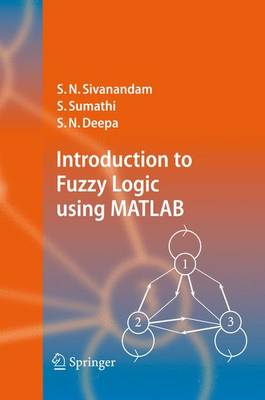•# Introduction to Fuzzy Logic using MATLAB (Paperback)

(author), (author), (author)
£99.99
Paperback 430 Pages / Published: 14/10/2010
• We can order this

Usually dispatched within 3 weeks

This book provides a broad-ranging, but detailed overview of the basics of Fuzzy Logic. The fundamentals of Fuzzy Logic are discussed in detail, and illustrated with various solved examples. The book also deals with applications of Fuzzy Logic, to help readers more fully understand the concepts involved. Solutions to the problems are programmed using MATLAB 6.0, with simulated results. The MATLAB Fuzzy Logic toolbox is provided for easy reference.

Publisher: Springer-Verlag Berlin and Heidelberg GmbH & Co. KG
ISBN: 9783642071447
Number of pages: 430
Weight: 676 g
Dimensions: 235 x 155 x 22 mm
Edition: Softcover reprint of hardcover 1st ed. 2007

MEDIA REVIEWS

From the reviews:

"This book constitutes a comprehensive and didactically appealing introduction to the essentials of fuzzy sets, fuzzy logic and their applications. The exposition of the material is presented in the commonly encountered computational framework of MATLAB. ... The didactic value of the book is very good. ... Examples and exercises are definitely a strong feature of the book ... . Overall, the book can be regarded as an excellent introductory text to all newcomers to fuzzy sets interested in their fundamentals and applications." (Witold Pedrycz, Zentralblatt MATH, Vol. 1109 (11), 2007)